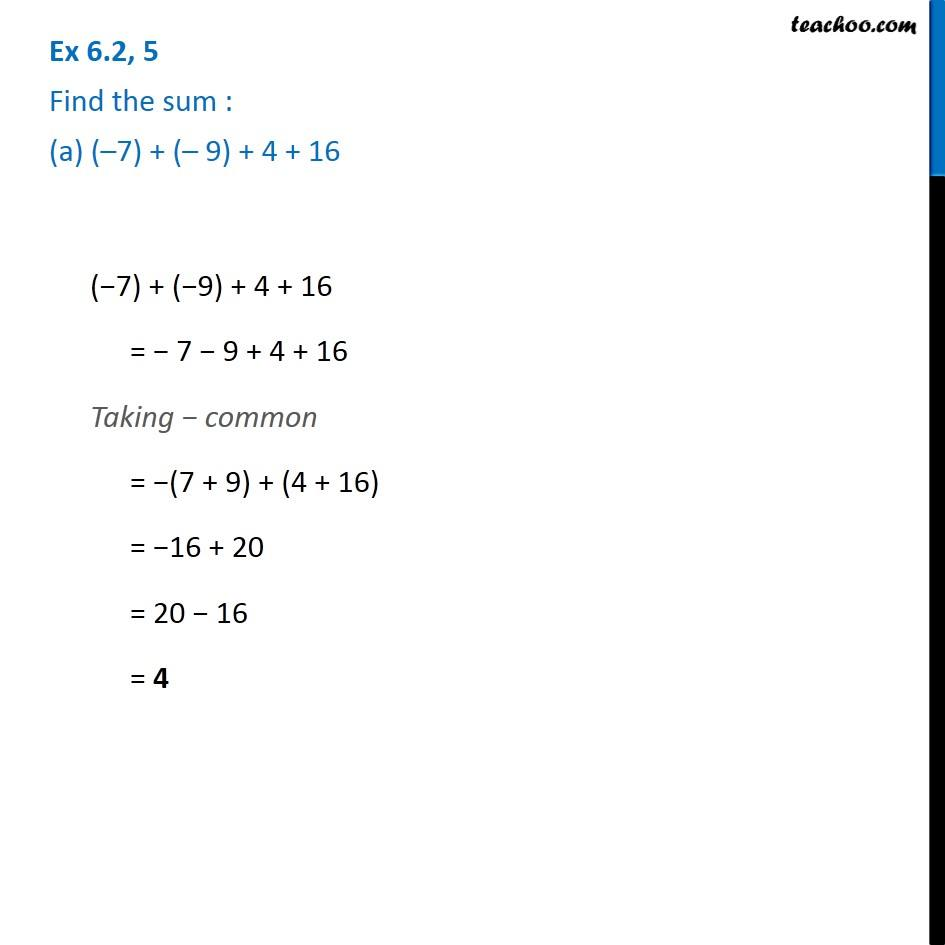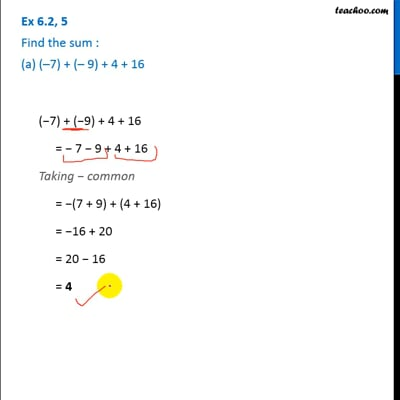Ex 6.2

Chapter 6 Class 6 Integers
Serial order wiseThis video is only available for Teachoo black users

### Transcript

Ex 6.2, 5 Find the sum : (a) ( 7) + ( 9) + 4 + 16 ( 7) + ( 9) + 4 + 16 = 7 9 + 4 + 16 Taking common = (7 + 9) + (4 + 16) = 16 + 20 = 20 16 = 4